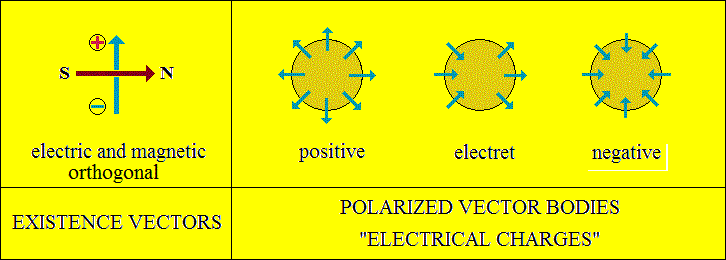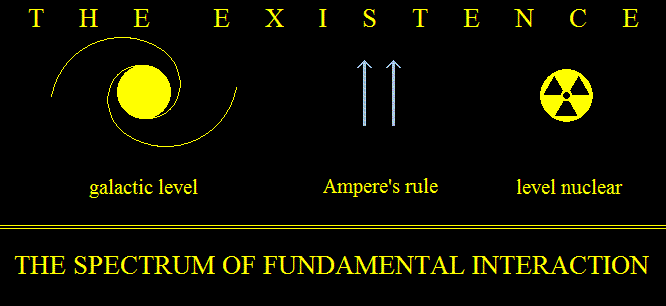Electrical charges, fundamental forces, electromagnetism and gravity.
Existence is energy, are vectori interactions.
The energy, the fundamental force, is the existence vector.
The vector as image is the symbol of the demonstrated properties in interactions.
The nature of these properties is inaccessible to knowledge.
So, the positive and negative electrical charges, south and north magnetic poles are vector polarities.With their properties, the vectors form currents with electromagnetic properties.
Electromagnetic currents, in turn, foment centripetal and centrifugal interactions,
according to Ampere's rule. Such interactions are the forces we find from the astronomical level
to the atomic level in the form of "gravity" or radiation.
Gravity is a vector interaction (electromagnetic).
It follows that a centripetal interaction with astronomical sizes, so space,
is perpetual compressed centripetal, with all its content.
This phenomenon is gravity.
This interpretation explains the phenomena observed:
Extended magnetic and electric fields, stratified pressure, energy, rotation, etc.
Consequences of vector interactions
1) Dimensions of interactions represent space.
2) The form of interactions represent matter (atoms, planets, stars).
3) The sense, the order of the interactions, only from the past toward future,
is the measure named time.
Attention! Space and time do not exist!
Space and time are the dimensions of energy, of interaction!
Without energy, neither time nor space exists!
The integer is a composition
Interaction consists of interactions (elementary, fundamental)
Space consists of spaces.
Time is composed of times.
The shape is made up of shapes.